Density 2

What is the density of a piece of driftwood that has the mass of 25 grams and a volume is 30 cm to the 3rd power. And how do I figure it out?

Result

d =  0.833 g/cm3

Solution:Leave us a comment of example and its solution (i.e. if it is still somewhat unclear...):Be the first to comment!Next similar examples:

1. CakesOn the bowl were a few cakes. Jane ate one-third of them, Dana ate a quarter of those cakes that remained. a) What part (of the original number of cakes) Dana ate? b) At least how many cakes could be (initially) on thebowl?
2. Brick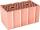Brick weight before drying is 5.3 kg. After drying, the bricks is 1 kg lighter and after firing is 0.7 kg lighter than after drying. What is the weight of the brick after drying and firing?
3. CagesHonza had three cages (black, silver, gold) and three animals (guinea pig, rat and puppy). There was one animal in each cage. The golden cage stood to the left of the black cage. The silver cage stood on the right of the guinea pig cage. The rat was in the
4. Line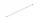How many parts of line divide 5 (different) points that lie on it?
5. Flood waterFlood waters in some US village meant that the homes had to evacuate 364 people. 50 of them stayed at elementary schools, 59 them slept with their friends and others went to relatives. How many people have gone to relatives?
6. 3 children3 children had to divide 4 pounds is candy. How much candy did each child get?
7. Doses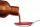A child is to receive a dose of 0.5 teaspoon of cough medicine every 12 hours. if the bottle contains 60 doses, how many days will the medicine last?
8. Expressions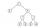Find out value of expressions if a = -1, b =2: x=b - 2a - ab y=a3 - b2 - 2ab z=a2 b3 - a3 b2 w=a + b + a3 - b2Added together and write as decimal number: LXVII + MLXIV
10. If-then equationIf 5x - 17 = -x + 7, then x =
11. Math classificationIn 3A class are 27 students. One-third got a B in math and the rest got A. How many students received a B in math?
12. Foot in busIt was 102 people on the bus. 28 girls had two dogs. A 11 girls had one dog. At the next stop seceded 5 dogs (even with their owners). They got two boys together with three dogs. The bus drove one driver. How many foot were in bus?
13. Circles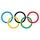For the circle c1(S1; r1=146 cm) and c2(S2; r2 = 144 cm) is distance of centers |S1S2| = 295 cm. Determine the distance between the circles.
14. One frame5 picture frames cost € 12 more than three frames. How much cost one frame?
15. Eq1Solve equation: 4(a-3)=3(2a-5)
16. The resultHow many times I decrease the number 1632 to get the result 24?
17. Valid numberRound the 453874528 on 2 significant numbers.